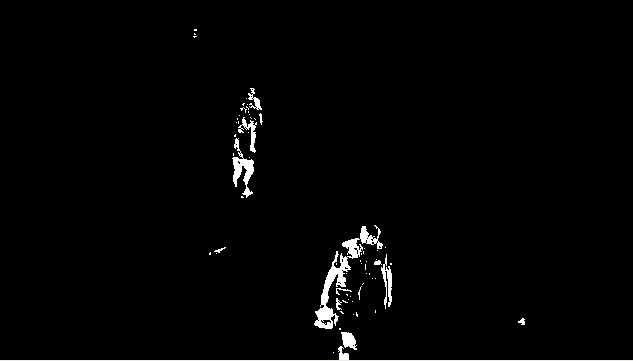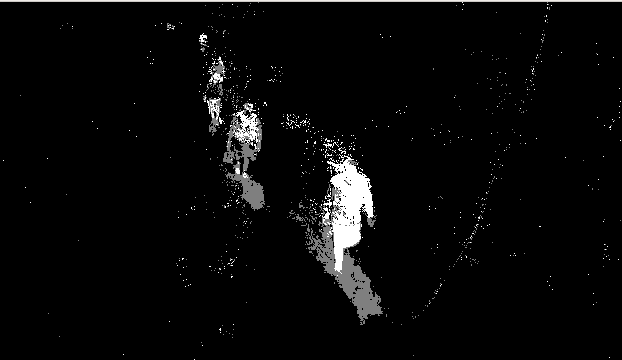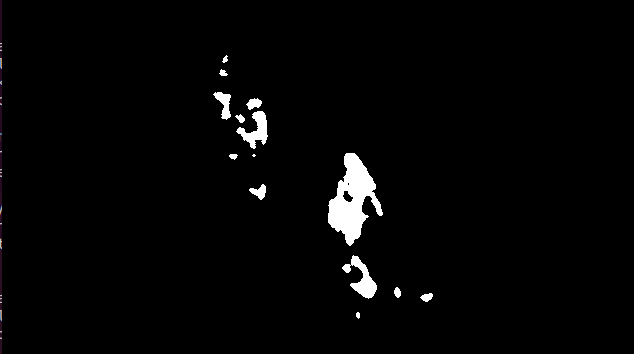Open in App
Not now

# Python OpenCV – Background Subtraction

• Last Updated : 03 Jan, 2023

Background Subtraction is one of the major Image Processing tasks. It is used in various Image Processing applications like Image Segmentation, Object Detection, etc. OpenCV provides us 3 types of Background Subtraction algorithms:-

• BackgroundSubtractorMOG
• BackgroundSubtractorMOG2
• BackgroundSubtractorGMG

Normally, we can perform background Subtraction using matrix subtraction, i.e, just subtracting the static frame from the video. But this has a lot of drawbacks. It is a very less efficient algorithm for Background subtraction because it does not update itself. This problem is being handled by the Background Subtraction algorithms provided by OpenCV.

## Using BackgroundSubtractorMOG

To use BackgroundSubtractorMOG we can use

`cv2.bgsegm.createBackgroundSubtractorMOG()`

Then we can apply it using the “apply” method on each frame of the video. Consider the below example for a better understanding of the topic.

Example:

 `import` `numpy as np``import` `cv2`` ` `cap ``=` `cv2.VideoCapture(``'sample.mp4'``)`` ` `# initializing subtractor ``fgbg ``=` `cv2.bgsegm.createBackgroundSubtractorMOG() `` ` `while``(``1``):``    ``ret, frame ``=` `cap.read()       `` ` `    ``# applying on each frame``    ``fgmask ``=` `fgbg.``apply``(frame)  `` ` `    ``cv2.imshow(``'frame'``, fgmask)``    ``k ``=` `cv2.waitKey(``30``) & ``0xff``    ``if` `k ``=``=` `27``:``        ``break`` ` `cap.release()``cv2.destroyAllWindows()`

Output :## Using BackgroundSubtractorMOG2

In the previous subtractor worked fairly well but in real-world situations, there is also a presence of shadows. In BackgroundSubtractorMOG2, we can also detect shadows and in the output of the following code, it’s clearly seen. To apply BackgroundSubtractorMOG2, use

`cv2.createBackgroundSubtractorMOG2()`

Example:

 `import` `numpy as np``import` `cv2`` ` `cap ``=` `cv2.VideoCapture(``'sample.mp4'``)`` ` `# initializing subtractor ``fgbg ``=` `cv2.createBackgroundSubtractorMOG2()`` ` `while``(``1``):``    ``ret, frame ``=` `cap.read()``  ` `    ``# applying on each frame``    ``fgmask ``=` `fgbg.``apply``(frame)`` ` `    ``cv2.imshow(``'frame'``, fgmask)  ``    ``k ``=` `cv2.waitKey(``30``) & ``0xff``    ``if` `k ``=``=` `27``:``        ``break`` ` `cap.release()``cv2.destroyAllWindows()`

Output :## Using BackgroundSubtractorGMG

This algorithm combines statistical background image estimation and per-pixel Bayesian segmentation. It employs a probabilistic foreground segmentation algorithm that identifies possible foreground objects using Bayesian inference. To use BackgroundSubtractorGMG, use

`cv2.bgsegm.createBackgroundSubtractorGMG()`

Note: We will get a black window during first few frames.

 `import` `numpy as np``import` `cv2`` ` `cap ``=` `cv2.VideoCapture(``'sample.mp4'``)`` ` `kernel ``=` `cv2.getStructuringElement(cv2.MORPH_ELLIPSE, (``3``, ``3``))`` ` `# initializing subtractor ``fgbg ``=` `cv2.bgsegm.createBackgroundSubtractorGMG()`` ` `while``(``1``):``    ``ret, frame ``=` `cap.read()`` ` `    ``# applying on each frame``    ``fgmask ``=` `fgbg.``apply``(frame)`` ` `    ``fgmask ``=` `cv2.morphologyEx(fgmask, cv2.MORPH_OPEN, kernel)     `` ` `    ``cv2.imshow(``'frame'``, fgmask)``    ``k ``=` `cv2.waitKey(``30``) & ``0xff``    ``if` `k ``=``=` `27``:``        ``break`` ` `cap.release()``cv2.destroyAllWindows()`

Output :My Personal Notes arrow_drop_up